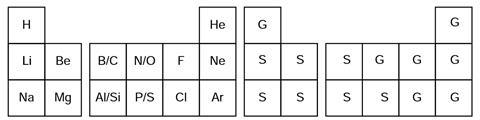Are three dimensions, one too many?

Explore Flatlandia, and convert what we know about our three-dimensional elements into a two-dimensional space.

## Questions

The periodic system of the elements in our three-dimensional world is based on the four electron quantum numbers n = 1,2,3…, l = 0, 1…, n-1; ml = 0, ±1, ±2 …, ±l; and ms = ±1⁄2. Let us move to Flatlandia.

It is a two-dimensional world where the periodic system of the elements is based on three electron quantum numbers: n = 1,2,3…; m = 0, ±1, ±2…, ±(n-1); and ms = ±1⁄2. m plays the combined role of l and ml of the three-dimensional worlds (ie s,p,d,… levels are related to m).

The following tasks and the basic principles relate to this two-dimensional Flatlandia where the chemical and physical experience obtained from our common three-dimensional world are applicable.

1. Draw the first four periods of the Flatlandian Periodic Table of the elements. Use the atomic number (Z) as the symbol of the element. Number the elements according to their nuclear charge. Give the electron configuration of each element.
2. Draw the hybrid orbitals of the elements with n=2. Which element is the basis for organic chemistry in Flatlandia? Give the Flatlandian analogues for ethane, ethene and cyclohexane. What kind of aromatic ring compounds are possible in Flatlandia?
3. Which rules in Flatlandia correspond to the octet and 18-electron rules in the three-dimensional world?
4. Predict graphically the trends in the first ionisation energies of the Flatlandian elements with n=2. Show graphically how the electronegativities of the elements increase in the Flatlandian Period Table.
5. Draw the molecular orbital energy diagrams of the neutral homonuclear diatomic molecules of the elements with n=2. Which of these molecules are stable in Flatlandia?
6. Consider simple binary compounds of the elements (n=2) with the lightest element (Z=1). Draw their Lewis structures, predict geometries and propose analogues for them in the three-dimensional world.
7. Consider elements with n<3. Propose an analogue and write the chemical symbol from our three-dimensional world for each Flatlandian element. On the basis of this chemical and physical analogy, predict which two-dimensional elements are solid, liquid or gas at the normal pressure and temperature.

### Question one - The Flatlandian periodic table:### Question two - Hybrid orbitals:There are no aromatic ring compounds.

### Question four - The ionisation energies and the trends in electronegativity### Question five - The molecular orbital diagram of the homonuclear X2 molecules### Question six - The Lewis structures and geometries### Question seven - The three dimensional analogues and the states of Flatlandian elements